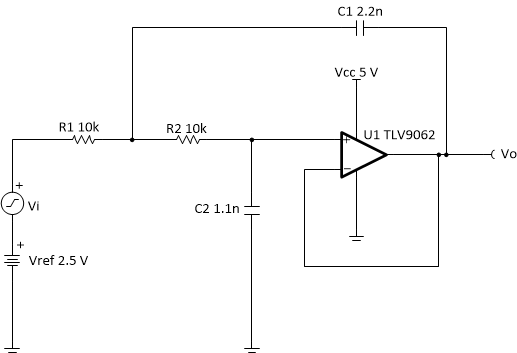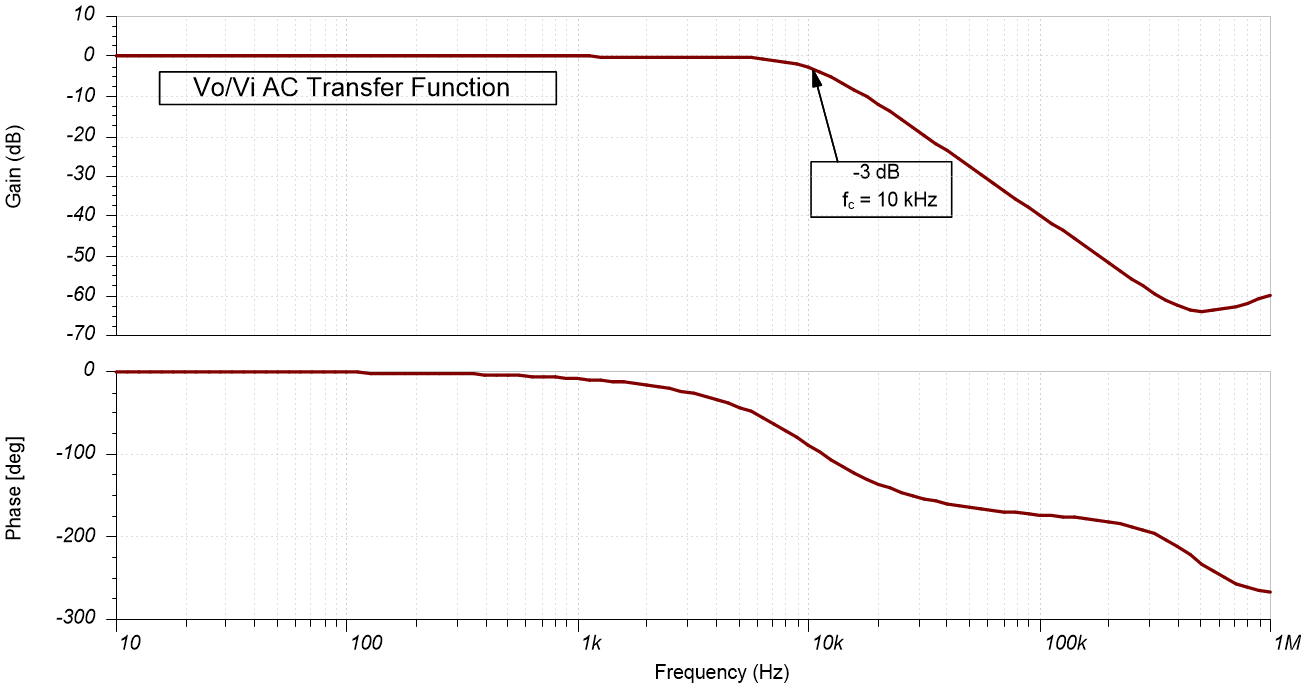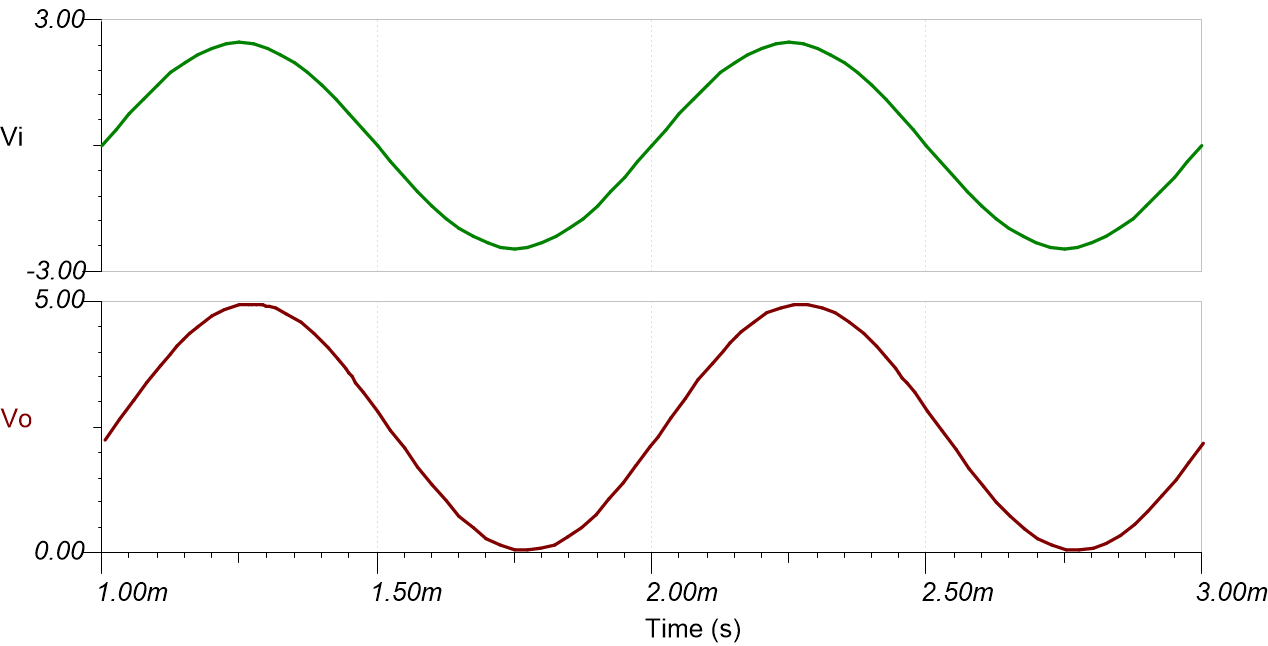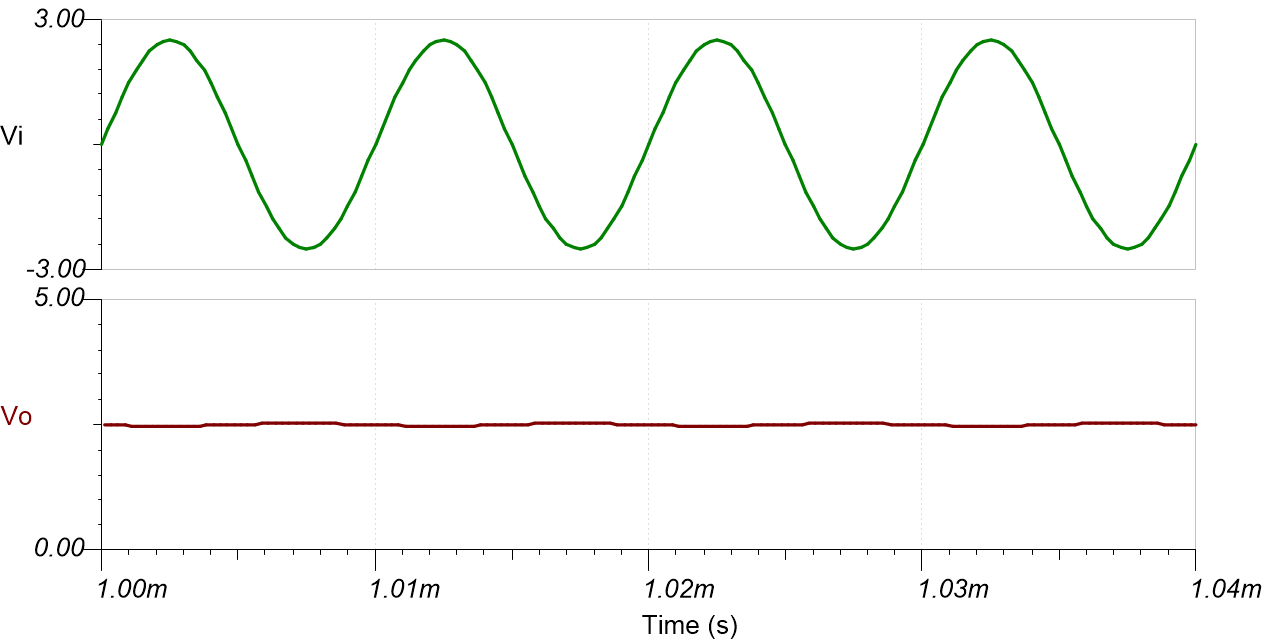SBOA226 June   2021

Input Output Supply
ViMin ViMax VoMin VoMax Vcc Vee
–2.45V +2.45V 0.05V 4.95V 5V 0V
Gain Cutoff Frequency (fc) Vref
1V/V 10kHz 2.5V

Design Description

The Butterworth Sallen-Key low-pass filter is a second-order active filter. Vref provides a DC offset to accommodate for single-supply applications. A Sallen-Key filter is usually preferred when small Q factor is desired, noise rejection is prioritized, and when a non-inverting gain of the filter stage is required. The Butterworth topology provides a maximally flat gain in the pass band.Design Notes

1. Select an op amp with sufficient input common-mode range and output voltage swing.
2. Add Vref to bias the input signal to meet the input common-mode range and output voltage swing.
3. Select the capacitor values first since standard capacitor values are more coarsely subdivided than the resistor values. Use high-precision, low-drift capacitor values to avoid errors in fc.
4. To minimize the amount of slew-induced distortion, select an op amp with sufficient slew rate (SR).

Design Steps

The first step is to find component values for the normalized cutoff frequency of 1 radian/second. In the second step the cutoff frequency is scaled to the desired cutoff frequency with scaled component values.

The transfer function for second order Sallen-Key low-pass filter is given by:

$\text{H (s)}=\frac{\frac{1}{{R}_{1}×{R}_{2}×{C}_{1}×{C}_{2}}}{{s}^{2}+s\left(\frac{1}{{R}_{1}×{C}_{1}}+\frac{1}{{R}_{2}×{C}_{1}}\right)+\frac{1}{{R}_{1}×{R}_{2}×{C}_{1}×{C}_{2}}}$
$\text{H(s)}=\frac{{a}_{0}}{{s}^{2}+{a}_{1}×s+{a}_{0}}$

Here,

1. Set normalized values of R1 and R2 (R1n and R2n) and calculate normalized values of C1 and C2 (C1n and C2n) by setting wc to 1 radian/sec (or fc = 1 / (2 × π) Hz). For the second-order Butterworth filter, (see the Butterworth Filter Table in the Active Low-Pass Filter Design Application Report).
2. Scale the component values and cutoff frequency. The resistor values are very small and capacitors values are unrealistic, hence these have to be scaled. The cutoff frequency is scaled from 1 radian/sec to w0. If m is assumed to be the scaling factor, increase the resistors by m times, then the capacitor values have to decrease by 1/m times to keep the same cutoff frequency of 1 radian/sec. If the cutoff frequency is scaled to be w0, then the capacitor values have to be decreased by 1 / wo. The component values for the design goals are calculated in steps 3 and 4.
Equation 1.
Equation 2. ${C}_{1}=\frac{{C}_{1n}}{m×{\omega }_{0}}=\frac{1.414}{m×{\omega }_{0}}F$
Equation 3. ${C}_{2}=\frac{{C}_{2n}}{m×{\omega }_{0}}=\frac{0.707}{m×{\omega }_{0}}F$
3. Set R1 and R2 values:
Equation 4. ${R}_{1}=\left({R}_{1n}×m\right)=10k\Omega$
Equation 5. ${R}_{2}=\left({R}_{2n}×m\right)=10k\Omega$
4. Calculate C1 and C2 based on m and w0.
5. Calculate the minimum required GBW and SR for fc.

The TLV9062 device has a GBW of 10MHz and SR of 6.5V/µs, so the requirements are met.

Design Simulations

AC Simulation ResultsTransient Simulation Results

The following image shows the filter output in response to 5-Vpp, 1-kHz input signal (gain = 1V / V).The following image shows the filter output in response to 5-Vpp, 100-kHz input signal (gain = 0.01 V/V).Design References

1. See Analog Engineer's Circuit Cookbooks for TI's comprehensive circuit library.
2. SPICE Simulation File SBOC598.
3. TI Precision Labs.
4. Active Low-Pass Filter Design Application Report

Design Featured Op Amp

TLV9062
Vss 1.8V to 5.5V
VinCM Rail-to-Rail
Vout Rail-to-Rail
Vos 0.3mV
Iq 538µA
Ib 0.5pA
UGBW 10MHz
SR 6.5V/µs
#Channels 1, 2, 4
www.ti.com/product/TLV9062

Design Alternate Op Amp

TLV316 OPA325
Vss 1.8V to 5.5V 2.2V to 5.5V
VinCM Rail-to-Rail Rail-to-Rail
Vout Rail-to-Rail Rail-to-Rail
Vos 0.75mV 0.150mV
Iq 400µA 650µA
Ib 10pA 0.2pA
UGBW 10MHz 10MHz
SR 6V/µs 5V/µs
#Channels 1, 2, 4 1, 2, 4
www.ti.com/product/TLV316 www.ti.com/product/OPA325